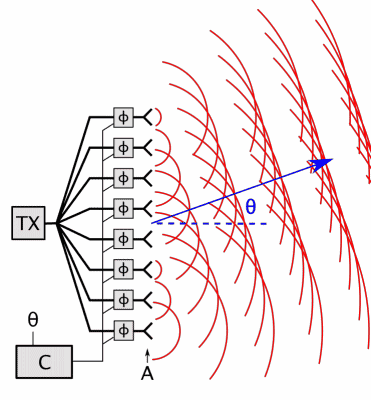# How would one calculate the distance and bearing of a signal source?

• I
KevinTOC
Basically, I'm wondering how you can essentially determine the bearing of a signal source, if you know its signal strength.

assuming you're in a vacuum, and you have a beacon transmitting a signal, and you know its signal strength. How could you find out the bearing (direction) it's at, if you know its signal strength, and if which way you're facing is 0°?

I've tried figuring it out myself, but I just can't get the final stuff:

Assuming the signal strength is 100k
The inverse square law says intensity = 1/distance^2 Therefore, Distance = sqrt(1/Intensity)
Therefore, distance (D) would be: sqrt(1/100k) = 0.003162
The distance is the Hypotenuse (a)
Therefore, c (the shortest side) = a/2 since in a right angled triangle, the hypotenuse is always double as long as the shortest side. Therefore, c = 0.001581
b
(The last side) is therefore sqrt(0.003162^2-0.001581^2) = 0.002738
The area of the triangle is A = (b*c)/2 = 0.000002 (2 x 10^-6)

The part I'm stuck on, is how to calculate angle ca? sin (0.001581/0.003162) Gets the angle 0.479426° Which doesn't seem right, since according to Google, it's supposed to be 53.14°

(If this is in the wrong forum category, I'm sorry.)

Homework Helper
Gold Member
intensity = 1/distance^2
The inverse square law is actually: I ∝1/D2, where ∝ means "is proportional to". To know the recveived intensity,[meant to say "distance"] you need the [received intensity], source intensity and the propagation model (spherical, cylindrical..). You also need to add some kind of diagram to clarify your scenario. Do you have multiple receivers?

Last edited:
Homework Helper
Therefore, c (the shortest side) = a/2 since in a right angled triangle, the hypotenuse is always double as long as the shortest side.

That's not true at all!

If we have an angle less than 45 degrees, then the opposite of that angle will be the shortest side. Since that angle can be anything in the range ##0^\circ < \theta < 45^\circ##, the ratio opposite/hypotenuse ##= \sin(\theta)## can be anything in the range ##0 < \sin(\theta) < 0.7071067## ... It definitely is not required to be 0.5.

Also this is wrong:
The inverse square law says intensity = 1/distance^2 Therefore, Distance = sqrt(1/Intensity)

As @lewando points out, the inverse square law says that intensity is proportional to ##1/d^2##, not equal. So if you know that the intensity is 1/100 of the intensity at, say, 2 m, then you know that your distance is ##\sqrt{100}## or 10 times as far as 2 m. In other words, it means you are 20 m from the source. Notice that to do that calculation, you need something to compare with, such as the intensity at a different known distance.

You can also calculate the distance if you know the total emitted power and the shape into which that power is being emitted. For instance, if you assume that it is spread out over a hemisphere, then you divide the total power by the area hemisphere of radius ##r## to get the power per unit area (intensity) at distance ##r## from the source.

But even once you know the distance, that doesn't answer your question. If I tell you something is 100 m away from you, does that identify only one possible location? Is there only one point in space which is 100 m from you?

With multiple power measurements, the problem is solvable.

Mentor
•Klystron
Homework Helper
You need to use a directional antenna to find the bearing.

https://en.m.wikipedia.org/wiki/Directional_antenna

That's the standard approach. But if you are able to determine the distance to multiple receive points based on power measurements, you can geolocate the source that way as well.

Mentor
Yes, of course. I didn't know that was an option it seemed the OP wanted to know how to do from a single point.

It wasn't mentioned by the OP but I've been involved in more than one project professionally to do just that kind of estimation, so it's of personal interest